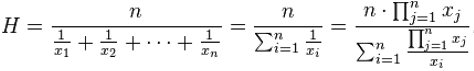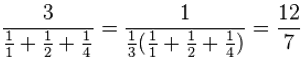Altair® Panopticon

### Harmonic Mean

The harmonic mean gives equal weight to each data point, meaning that extreme outlier values will not impact the Harmonic Mean as much as it would an Arithmetic Mean.

Typically, it is appropriate for situations when the average of rates is desired. The Harmonic mean H of the positive real numbers x1, x2, ..., xn > 0 is defined to be:Sample 1:

As a simple example, the Harmonic mean of 1, 2, and 4 isor 1.7143

Sample 2:

Another example based on the number of hours worked per week:

The table shows the average working hours per week per employee (a rate). Each employee was only required to work 2000 hours but their working hours differs per week:

 Employee Total Hours Worked Average Working Hours Per Week Work Weeks Joy 2000 50 40 Thomas 2000 45 44.4444 Erick 2000 35 57.142857 John 2000 40 50

Employee working hours per week

The total number of working hours by all four employees is 8000 hours. The total number of work weeks is 191.59 weeks. The calculation to compute for the Harmonic mean is:

4/(1/50 + 1/45 + 1/35 + 1/40) = 41.7564 hours

A simple check of dividing 8000 hours by 41.76 will equal 191.59 which is the total number of weeks the employees worked.